# qsimulatR Package

In this vignette we provide basic usage examples of the qsimulatR package.

## A quantum state

The basis for the simulator is a quantum state, called qstate in qsimulatR realised as an S4 class. One can generate a qstate with a number of qubits as follows

x <- qstate(nbits=2)
x
   ( 1 )    * |00> 

Per default this state is in the computational basis with all qubits in the $$|0\rangle$$ state. The basis state can also be supplied to qstate as an optional argument, e.g.

CatInBox <- qstate(nbits=1, basis=c("|dead>", "|alive>"))
CatInBox
   ( 1 )    * |dead> 

and also the coefficients of the separate basis states can be set

CatInBox <- qstate(nbits=1, basis=c("|dead>", "|alive>"),
coefs=as.complex(c(1/sqrt(2), 1/sqrt(2))))
CatInBox
   ( 0.7071068 )    * |dead>
+ ( 0.7071068 )    * |alive> 

A qstate object can also be plotted. If no gate was applied yet, this is not very interesting

plot(x, qubitnames=c("bit1", "bit2"))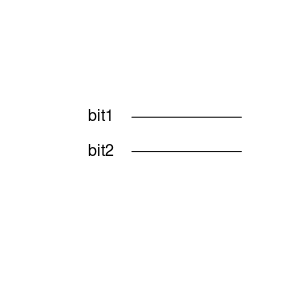## Applying single qubit gates

Next one can apply single qubit quantum gates to such a qstate, like for instance the Hadamard gate

x <- qstate(nbits=2)
y <- H(1) * x
y
   ( 0.7071068 )    * |00>
+ ( 0.7071068 )    * |01> 

where the argument to H is the qubit to which to apply the gate. in R we start counting with 1, which corresponds to the least significant bit (or the rightmost one in $$|00\rangle$$). H(1) returns an S4 object of class sqgate and the multiplication is overloaded.

Now, with a gate included, plotting the state represents the quantum circuit used to generate the state, for instance

plot(y)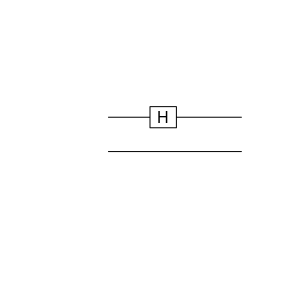Even if there is a list of predefined single qubit gates (H, X, Z, Y, Rz, Id, S, Tgate), any single qubit gate can be defined as follows

myGate <- function(bit) {
methods::new("sqgate", bit=as.integer(bit),
M=array(as.complex(c(1,0,0,-1)), dim=c(2,2)),
type="myGate")
}

From now on this gate can be used like the predefined ones

z <- myGate(1) * y
z
   ( 0.7071068 )    * |00>
+ ( -0.7071068 )   * |01> 

The argument M must be a unitary $$2\times 2$$ complex valued matrix, which in this case is the third Pauli matrix predefined as Z.

### Truth Table

Sometimes it is useful to look at the quantum analogon of a truth table of a gate as it immediately shows how every qubit is influenced depending on the other qubits. It can provide a consistency check for a newly implemented gate, too. The truth table of a single qubit gate (here the X gate) can be obtained as follows

truth.table(X, 1)
  In1 Out1
1   0    1
2   1    0

## Multi-qubit gates

The most important two-qubit gate is the controlled NOT or CNOT gate. It has the truth table

truth.table(CNOT, 2)
  In2 In1 Out2 Out1
1   0   0    0    0
2   0   1    1    1
3   1   0    1    0
4   1   1    0    1

and can for instance be used as follows to generate a Bell state

z <- CNOT(c(1,2)) * y
z
   ( 0.7071068 )    * |00>
+ ( 0.7071068 )    * |11> 

Plotting the resulting circuit looks as follows

plot(z)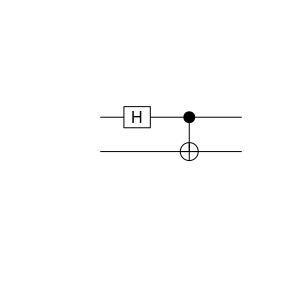The arguments to CNOT are the controll and target bit in this order. Again, arbitrary controlled single qubit gates can be generated as follows

CX12 <- cqgate(bits=c(1L, 2L), gate=X(2L))
z <- CX12 * y
z
   ( 0.7071068 )    * |00>
+ ( 0.7071068 )    * |11> 

which in this case is the CNOT gate again with control bit 1 and target bit 2. cqgate is a convenience function to generate a new S4 object of S4 class cqgate. Note that a similar function sqgate is available. The gate argument to cqgate can be any sqgate object.

Another widely used two-qubit gate is the SWAP gate

z <- SWAP(c(1,2)) * y
z
   ( 0.7071068 )    * |00>
+ ( 0.7071068 )    * |10> 
plot(z)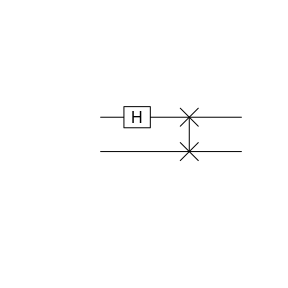The order of the bits supplied to SWAP is irrelevant, of course

z <- SWAP(c(2,1)) * y
z
   ( 0.7071068 )    * |00>
+ ( 0.7071068 )    * |10> 

Finally, there are also the controlled CNOT or Toffoli gate and the controlled SWAP gate available

x <- H(1) *(H(2) * qstate(3))
x
   ( 0.5 )  * |000>
+ ( 0.5 )  * |001>
+ ( 0.5 )  * |010>
+ ( 0.5 )  * |011> 
y <- CCNOT(c(1,2,3)) * x
y
   ( 0.5 )  * |000>
+ ( 0.5 )  * |001>
+ ( 0.5 )  * |010>
+ ( 0.5 )  * |111> 
plot(y)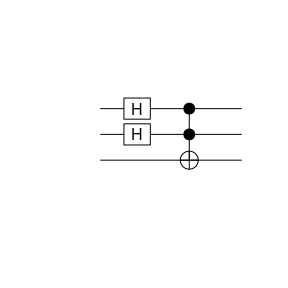The arguments to CCNOT is a vector of three integers. The first two integers are the control bits, the last one the target bit.

Similarly, the CSWAP or Fredkin gate

y <- CSWAP(c(1,2,3)) * x
y
   ( 0.5 )  * |000>
+ ( 0.5 )  * |001>
+ ( 0.5 )  * |010>
+ ( 0.5 )  * |101> 
plot(y)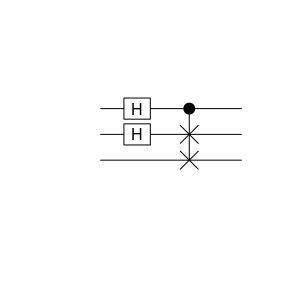## Multi-gate circuits as functions

You might want to use specific circuits of several gates more than once and therefore find it tedious to type it completely every time you use it. Defining a new gate combining all of the operations might not be as easy either. In these cases we find it convenient to define generating functions of the form

myswap <- function(bits){
function(x){
CNOT(bits) * (CNOT(rev(bits)) * (CNOT(bits) * x))
}
}

where an outer function takes any required information concerning the circuit. It then returns a function that applies given circuit to a state.

Our example above implements a SWAP using only CNOT gates as can be checked with the truth table:

truth.table(myswap, 2)
  In2 In1 Out2 Out1
1   0   0    0    0
2   0   1    1    0
3   1   0    0    1
4   1   1    1    1

We can plot the circuit in the way we are used to as well.

circuit <- myswap(1:2)
plot(circuit(qstate(2)))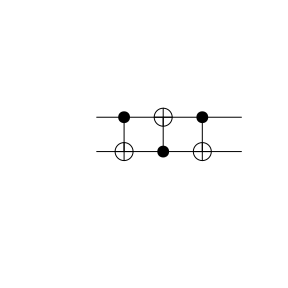## Measurements

At any point single qubits can be measured

res <- measure(y, 1)
summary(res)
Bit 1 has been measured 1 times with the outcome:
0:  0
1:  1 
plot(res$psi)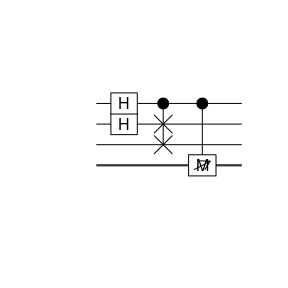In this case the third qubit is measured and its value is stored in res$value. The wave function of the quantum state collapses, the result of which is stored again as a qstate object in res$psi. Such a measurement can be repeated, say 1000 times, for qubit 3 rv <- measure(y, 3, rep=1000)$value
hist(rv, freq=FALSE, main="Probability for Qubit 3")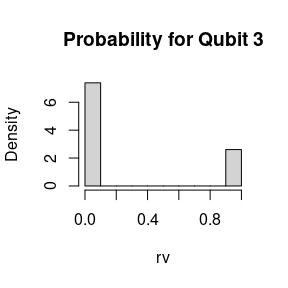where we read of from the wave function that the ratio should be $$3:1$$ for values $$0$$ and $$1$$, which is well reproduced.

# Export ot Qiskit

IBM provides quantum computing platforms, which can be freely used. However, one needs to programme in python using the qiskit package. For convenience, qsimulatR can export a circuit translated to qiskit

filename <- paste0(tempdir(), "/circuit.py")
export2qiskit(y, filename=filename)

which results in the following file

cat(readLines(filename), sep = '\n')
# automatically generated by qsimulatR
qc = QuantumCircuit(3)
qc.h(1)
qc.h(0)
qc.cswap(0, 1, 2)

which can be used at quantum-computing.ibm.com to run on real quantum hardware. Note that the export functionality only works for standard quantum gates, not for customary defined ones.